# DIVISION

##### HELLO, WELCOME BACK TO CLASS

Division is a method of distributing a group of things into equal parts. It is a way of sharing into equal parts. Division is the opposite of multiplication. It is one of the four basic operations in arithmetic. The others are addition, subtraction and multiplication.

In this lesson we will be learning how to divide with 2 digits and 3-digit number

Worked Examples: Divide

1. 618 by 12. b)  8268 by 15       c) 4573 by 52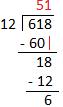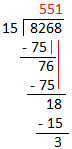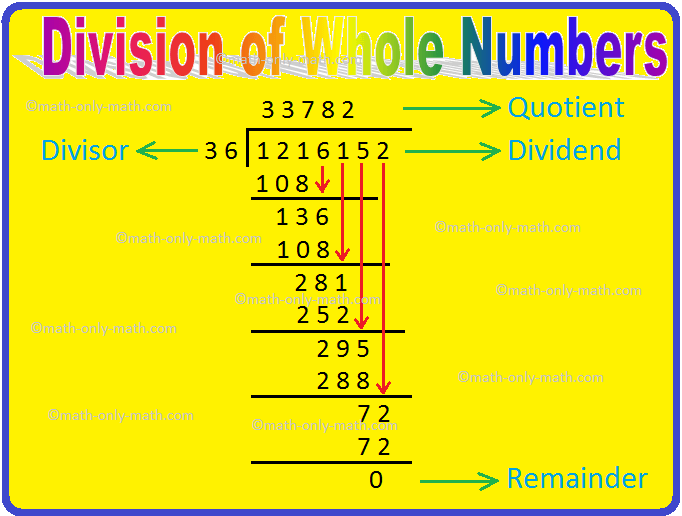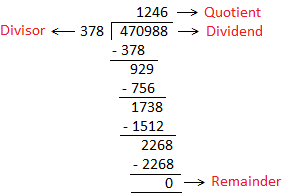ACTIVITY

Divide and verify the result:

(i) 5184 ÷ 16

(ii) 1331 ÷ 11

(iii) 4956 ÷ 14

(iv) 8675 ÷ 17

(v) 8234 ÷ 18

(vi) 6932 ÷ 29

Word Problem on Division

If 9975 kg of wheat is packed in 95 bags, how much wheat will each bag contain?

Solution:

Since 95 bags contain wheat 9975 kg

Therefore, 1 bag contains wheat (9975 ÷ 95) kg

= 105 kg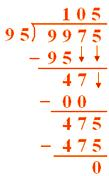ACTIVITIES

1. 89 people have been invited to a banquet. The caterer is arranging tables. Each table can seat 12 people. How many tables are needed?
2. A bus can hold 108 passengers. If there are 12 rows of seats on the bus, how many seats are in each row?
3. Mark baked 195 cookies and divided them equally into 13 packs. How many cookies did Mark put in each packet?
4. There are 1025 students in a school containing 25 sections. If in each section there are equal number of students, find their number in each section
5. The product of two numbers is 4131. If one of them is 27, find the other.

Your Opinion Matters! Quickly tell us how to improve your Learning Experience HERE